NEET  >  Test: Quantitative Analysis (NCERT)

# Test: Quantitative Analysis (NCERT) - NEET

Test Description

## 10 Questions MCQ Test - Test: Quantitative Analysis (NCERT)

Test: Quantitative Analysis (NCERT) for NEET 2023 is part of NEET preparation. The Test: Quantitative Analysis (NCERT) questions and answers have been prepared according to the NEET exam syllabus.The Test: Quantitative Analysis (NCERT) MCQs are made for NEET 2023 Exam. Find important definitions, questions, notes, meanings, examples, exercises, MCQs and online tests for Test: Quantitative Analysis (NCERT) below.
Solutions of Test: Quantitative Analysis (NCERT) questions in English are available as part of our course for NEET & Test: Quantitative Analysis (NCERT) solutions in Hindi for NEET course. Download more important topics, notes, lectures and mock test series for NEET Exam by signing up for free. Attempt Test: Quantitative Analysis (NCERT) | 10 questions in 10 minutes | Mock test for NEET preparation | Free important questions MCQ to study for NEET Exam | Download free PDF with solutions
 1 Crore+ students have signed up on EduRev. Have you?
Test: Quantitative Analysis (NCERT) - Question 1

### 0.92 g of an organic compound was analysed by combustion method. The mass of the U-tube increased by 1.08 g. What is the percentage of hydrogen in the compound?

Detailed Solution for Test: Quantitative Analysis (NCERT) - Question 1

Increase in mass of U-tube = 1.08 g
Mass of water formed = 1.08 g
18gH20 = 2gH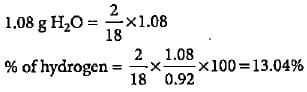Test: Quantitative Analysis (NCERT) - Question 2

### An organic compound gave 0.4655 g of CO2 on complete combustion. If the mass of the compound taken was 0.2115 g, what is the percentage of C in it?

Detailed Solution for Test: Quantitative Analysis (NCERT) - Question 2

Mass of CO2 formed = 0.4655 g
Mass of organic compound taken = 0.2115 g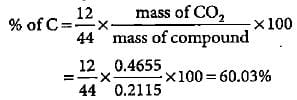Test: Quantitative Analysis (NCERT) - Question 3

### In Duma'smethod 0.52 g of an organic compound on combustion gave 68.6 mL N2 at 27oC and 76 mm pressure. What is the percentage of nitrogen in the compound?

Detailed Solution for Test: Quantitative Analysis (NCERT) - Question 3

V1 = 68.6 mL, P1 =. 756 mm, T1 = 300 K V2 = ?, P2 = 760 mm, T2 = 273 K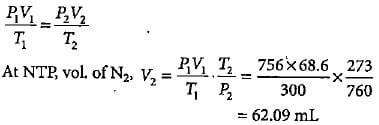Percentage of nitrogen in organic compound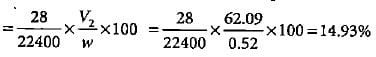Test: Quantitative Analysis (NCERT) - Question 4

In kjeldahl's method of estimation of nitrogen, nitrogen is quantitatively converted to ammonium sulphate. It is the treated with the standard solution of alkali. The nitrogen which is present is estimated as:

Detailed Solution for Test: Quantitative Analysis (NCERT) - Question 4

When treated with standard solution of alkali nitrogen is liberated as NH3 which is absorbed in H2SO4 to get percentage of nitrogen.

Test: Quantitative Analysis (NCERT) - Question 5

In Carius method of estimation of halogen, 0.15 g of an organic compound gave 0.12 g of AgBr. What is the percentage of bromine in the compound?

Detailed Solution for Test: Quantitative Analysis (NCERT) - Question 5

Molar mass of AgBr = 108 + 80 = 188 g mol-1
Percentage of Br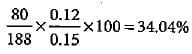Test: Quantitative Analysis (NCERT) - Question 6

2.18 g of an organic compound containing sulphur produces 1.02g of BaSO4. Thepercentage of sulphur in the compound is

Detailed Solution for Test: Quantitative Analysis (NCERT) - Question 6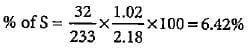Test: Quantitative Analysis (NCERT) - Question 7

1.6 g of an organic compound gave 2.6 g of magnesium pyrophosphate. The percentage of phosphorus in the compound is

Detailed Solution for Test: Quantitative Analysis (NCERT) - Question 7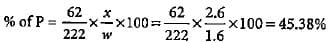Test: Quantitative Analysis (NCERT) - Question 8

Match the column I with column II in which formula for estimation of an element is given and mark the appropriate choice.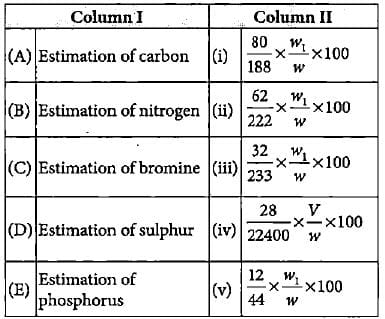Detailed Solution for Test: Quantitative Analysis (NCERT) - Question 8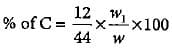(w1 = weight of CO2, w= weight of organic compound)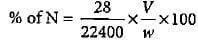(V = volume of N2 at STP, w = weight of organic compound)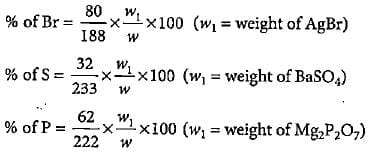Test: Quantitative Analysis (NCERT) - Question 9

The percentage of oxygen in heavy water is

Detailed Solution for Test: Quantitative Analysis (NCERT) - Question 9

In D2O percentage of deuterium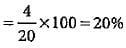% of oxygen - 100 - 20 = 80%

Test: Quantitative Analysis (NCERT) - Question 10

0.2 g of an organic compound contains C, H and O.On combustion, it yields 0.15 g CO2 and 0.12 g H2O.Ihe percentage of C, H and O respectively is

Detailed Solution for Test: Quantitative Analysis (NCERT) - Question 10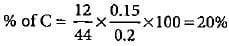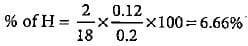% of O = 100 - (20 + 6.66) = 73.34%

Information about Test: Quantitative Analysis (NCERT) Page
In this test you can find the Exam questions for Test: Quantitative Analysis (NCERT) solved & explained in the simplest way possible. Besides giving Questions and answers for Test: Quantitative Analysis (NCERT), EduRev gives you an ample number of Online tests for practice

### How to Prepare for NEET

Read our guide to prepare for NEET which is created by Toppers & the best Teachers(Scan QR code)